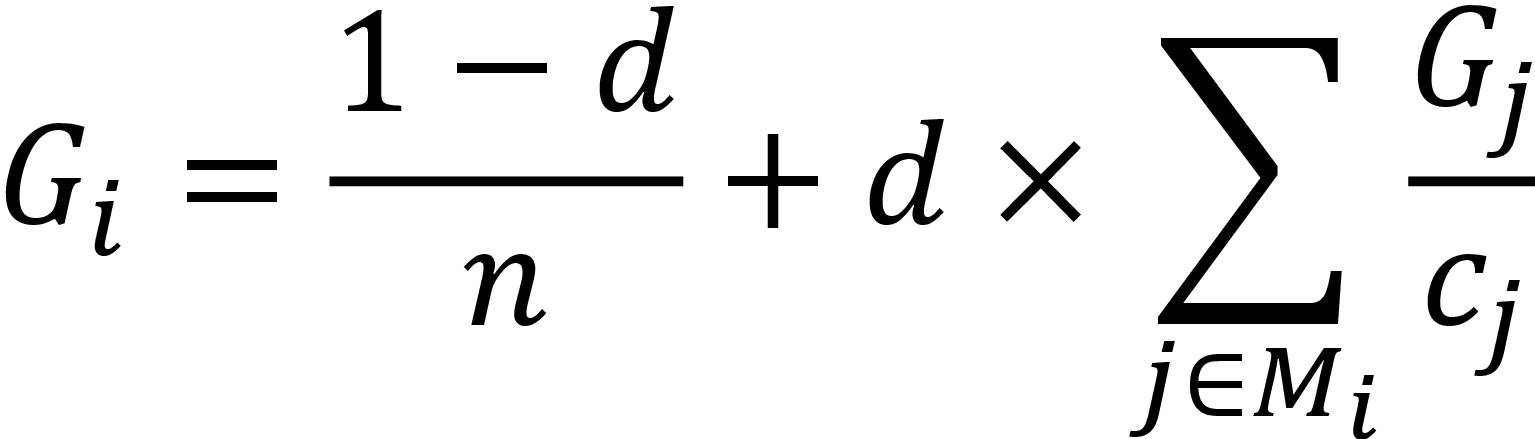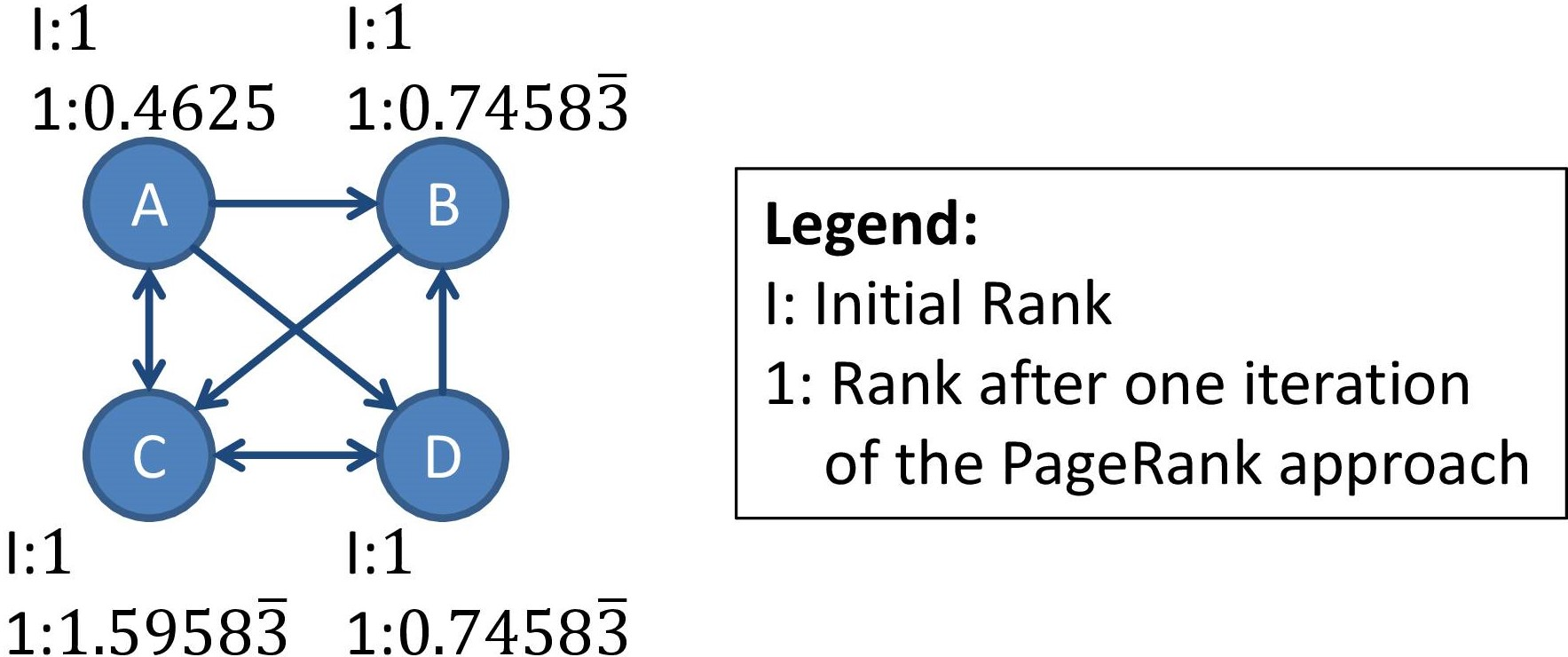Tutorial Webpage for

Lecture Cloud- and Web-Technologies

## Map/Reduce - PageRank

Your task is to develop a MapReduce-program for calculating the weights according to the PageRank approach.

The PageRank approach is still used today (besides other criteria) in search engines as basis for the ranking of websites. The input of the PageRank algorithm is a graph, where the (weighted) nodes represent the websites and the weighted edges between the nodes the links between the websites.

The weigths of the edges are after applying the PageRank approach as larger as more nodes (with large weights) are linked to this node. The weight G_i of a node i is calculated from the weights G_j of those nodes M_i, which are linked to the node i (i.e., which edges end in node i). If j (in M_i) is linked to c_j different nodes, then the weight of G_j is proportionally divided between these nodes. Hence one iteration of the PageRank approach can be described by the following formula for the determination of the new weight G_i of a node i:In the given formula, d is a damping factor and n the total number of nodes.

The PageRank approach is typically processed in a given number of iterations (and sometimes based on converging ranks). In this exercise we compute the ranks of given websites only in one iteration. The input data is given in the form i G_i M_i, where the nodes in M_i are separated by commas.

Example:#### Data

The following data of the file PageRankInput.txt is given:

## Editor

Please use the following editor for your exercise. You can run the content of the editor by clicking on the tab 'Run'...

## Output of Editor Content

The result of running the editor content is as follows:

## Output of the Solution

The output of the solution is as follows:

## Solution

Please have a look at the solution only after you have finished working on your own solution. Otherwise the learn effect is much less.